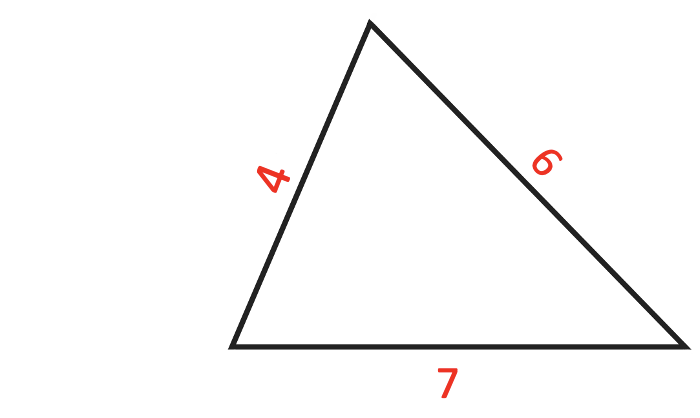# Problem 52574. Easy Sequences 8: Triangles with integer sides and prime perimeters

The triangle below is special.It has integer sides and a prime perimeter.
Given an integer "n" we want to know how many triangles can be formed such that the sides are integers and the perimeters are primes less than or equal to the nearest prime to "n".
The nearest prime number is defined as:
• "n" if "n" itself is prime;
• either the previous prime before or the next prime after "n", whichever has lesser distance to "n"; or
• the previous prime before "n" if the previous and next primes are equidistant to "n".
As an example, lets consider "n = 9". The nearest prime is 7 because 9 is equidistant between 7 and 11. The primes less than equal to 7 are [2 3 5 7]. There are no integral triangles that can be formed with perimeter 2. For 3 there is one, namely [1 1 1]. For 5 there is one, [1 2 2]. And for 7 there are 2, [1 3 3] and [2 2 3] . So, the total number of prime perimetered integral triangles that can be formed when n = 9 is 4.
NOTE: Rotations and reflections are irrelevant and counted only once.

### Solution Stats

75.0% Correct | 25.0% Incorrect
Last Solution submitted on May 29, 2023

### Community Treasure Hunt

Find the treasures in MATLAB Central and discover how the community can help you!

Start Hunting!